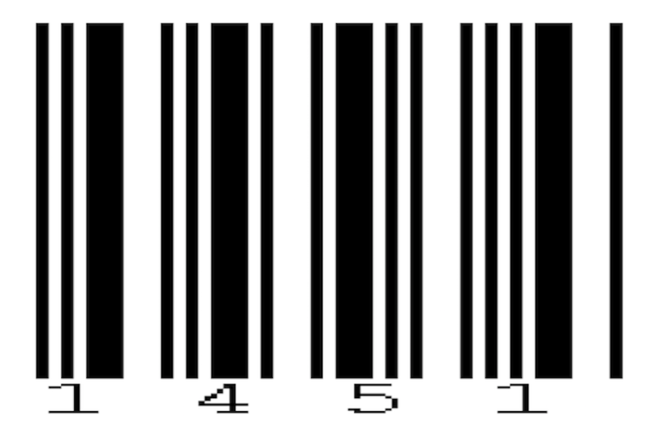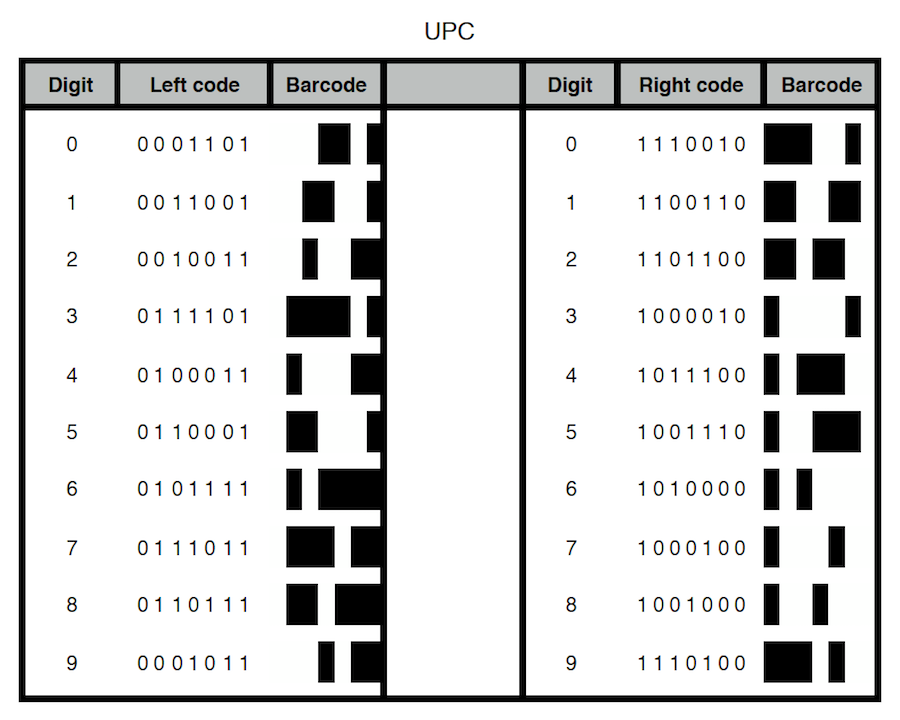2.20

## Weizmann Institute of ScienceThe Codabar barcode of 1451

# What are Barcodes?

A barcode is an image that uses lines of different thickness to represent information, usually, but not exclusively, digits.

It is designed to be read by a special scanner in order to input it to a computer in a quick, automatic way.

There are several advantages to the use of barcodes:

• When the price of an item changes, you only need to update the database in the computer, and not the items themselves.
• Their use greatly reduces errors, allowing easy inventory checks.
• It protects against customers who change the price labels.

There are two main types of barcode symbologies:
- Discrete – each digit is represented separately. There is a visual distinction between each digit. - Continuous – there is no clear distinction between the digits.

# Discrete barcodes

Here is the encoding of a discrete barcode symbology called ‘Codabar’.In this barcode each digit is encoded using: three narrow lines, a single wide line, two narrow gaps, and a single wide gap. For each digit these lines and gaps are placed in a different order.

The digit 0, for example, is encoded: narrow line, narrow gap, narrow line, narrow gap, narrow line, wide gap and wide line.

The digit 1, for example, is encoded: narrow line, narrow gap, narrow line, narrow gap, wide line, wide gap, narrow line. This method of encoding is called Codabar.

The image at the top of the page is the barcode of the number 1451, in Codabar.

# Continuous barcodes

Most countries use continuous barcodes. One of the methods for encoding continuous barcodes is called UPC (Universal Product Code). This method encodes a twelve-digit number and is referred to as UPC-12 to distinguish it from the six-digit UPC-E barcode. The encoding is done as follows: a starting symbol, six encoded digits, a middle symbol, six encoded digits and an end symbol. The start, middle and end symbols are known as: “Guard Bars”.

Here is an example of a UPC barcode:• The beginning and end symbols are two long narrow lines with a narrow gap between them.
• The middle symbol is a narrow gap followed by a narrow line, then a narrow gap, a narrow line and a final narrow gap.
• The digits in UPC are encoded into a sequence of lines and gaps in the following manner:

Two tables are used, each one having ten rows and seven columns:In each table, the rows indicate the coding of digits from 0 to 9. The difference between the two tables is that one table indicates the coding of the left-hand digits, while the other is for the right-hand ones. In each column the digit ‘0’ represents a gap, and ‘1’ represents a line. This way, the seven digits (0 or 1) in each row in the table make up a sequence of lines and gaps which are that digit’s code. If we take, for example, a sequence of three ‘1’s (‘111’), the line would be three times as thick as a line coded from a single ‘1’ digit (‘010’). Another example: ‘00’ stands for a double gap. A single gap is encoded with a single ‘0’.

Here is the UPC coding for 012345678912:Attached below are pdf files of the UPC and Codabar encodings.

If you’ve taken the trouble to look at a barcode on a product, you might find a barcode that looks like UPC, but has 13 digits instead of 12. This is because the last digit is what’s known as a checksum. We’ll take a look at checksums in the following step. In the meantime, I’d like to ask you to do me a favour. Take a look at some barcodes on everyday products and see if you can discover the symbology used. You can use this article to help.

Don’t forget to let us know what you found in the comments below.